# Intermediate Maths Solutions for Exercise 5(b) Product of Vectors

Intermediate mathematics IA Exercise 5(b) Product of Vectors textbook solutions are given.

These solutions are very easy to understand.

Please study the textbook lesson Product of Vectors very well.

Then observe the example problems and solutions given in the text book. Try them.

Observe the solutions given below. Try them in your own method.

You can see the solutions for text book Maths 1A

Functions

Exercise 1(a)

Exercise 1(b)

Exercise 1(c)

Mathematical Induction

Exercise 2(a)

Matrices

Exercise 3(a)

Exercise 3(b)

Exercise 3(c)

Exercise 3(d)

Exercise 3(e)

Exercise 3(f)

Exercise 3(g)

Exercise 3(h)

Exercise 3(i)

Exercise 4(a)

Exercise 4(b)

Product of Vectors

Exercise 5(a)

Exercise 5(b)

Exercise 5(c)

Trigonometric Ratios up to Transformations

Exercise 6(a)

Exercise 6(b)

Exercise 6(c)

Exercise 6(d)

Exercise 6(e)

Exercise 6(f)

Trigonometric Equations

Exercise 7(a)

Inverse Trigonometric Equations

Exercise 8(a)

Hyperbolic Functions

Exercise 9(a)

Properties of Triangles

Exercise 10(a)

Exercise 10(b)

Maths 1B textbook solutions

Locus

Exercise 1(a)

Transformation of axes

Exercise 2(a)

Three Dimensional Coordinates

Exercise 5(a)
Exercise 5(b)
Direction Cosines and Direction Ratios

Exercise 6(a)
Exercise 6(b)
The Plane

Exercise 7(a)
Limits and continuity

Exercise 8(a)

Exercise 8(b)

M

You can see text book solutions for Inter maths IIA

You can see solutions for Examination purpose

You can see text book solutions for Inter Maths 2b

1. Circle

3. Parabola

4. Ellipse

M

# Inter Maths solutions for Exercise 5(b) Product of Vectors

Product of Vectors – Exercise 5(b)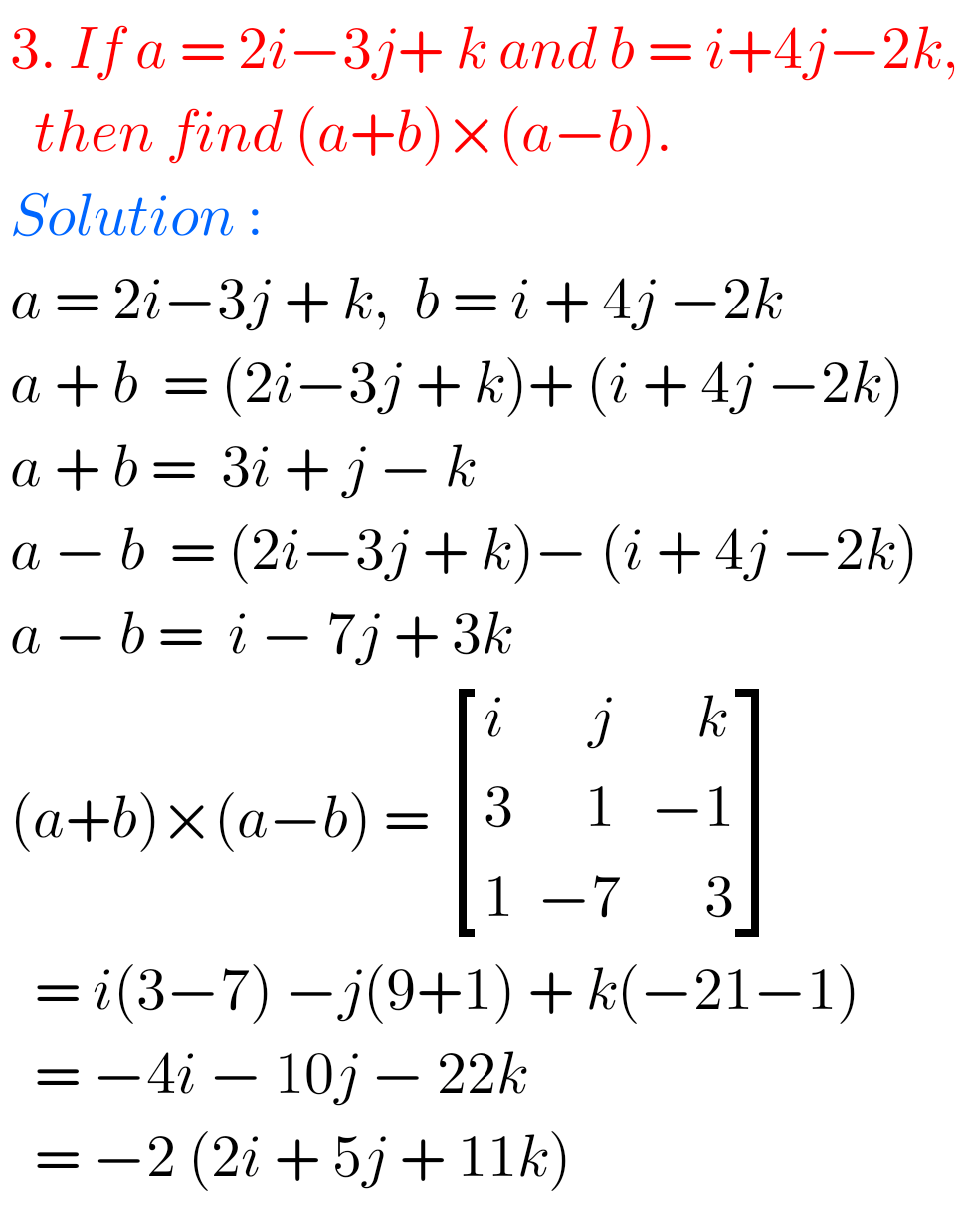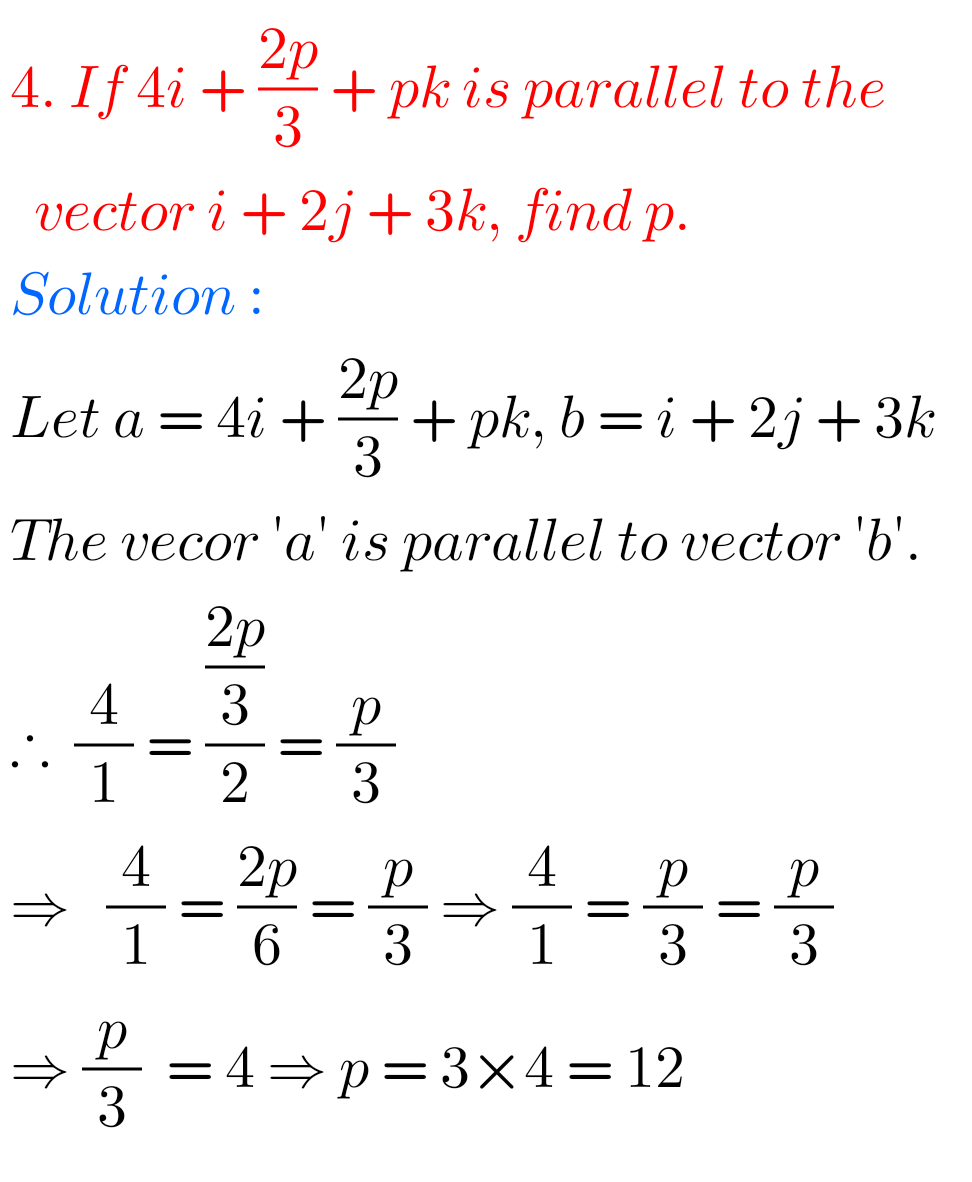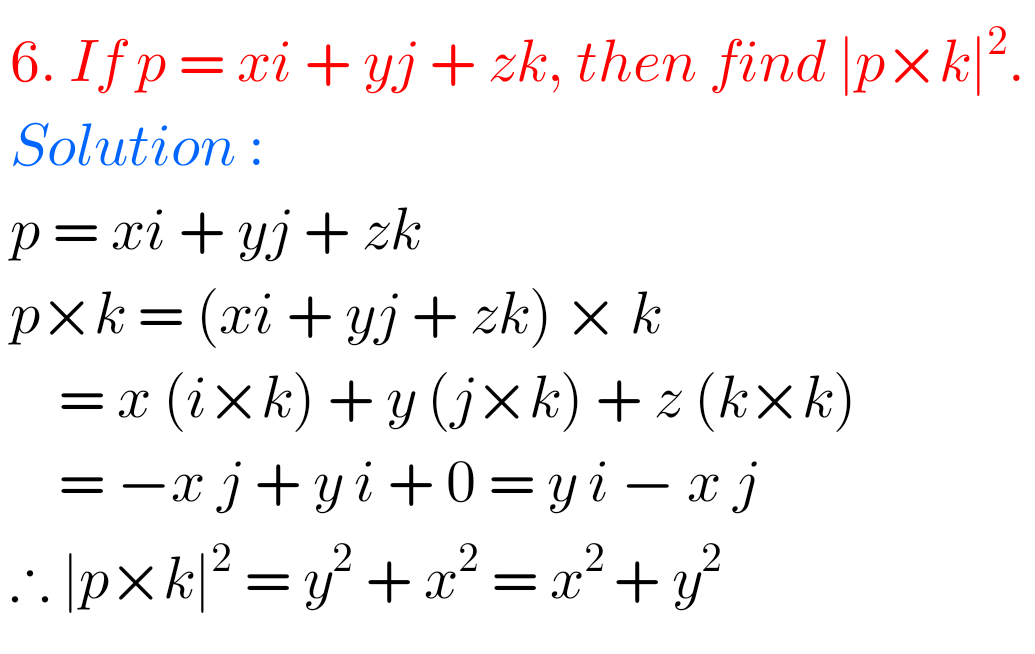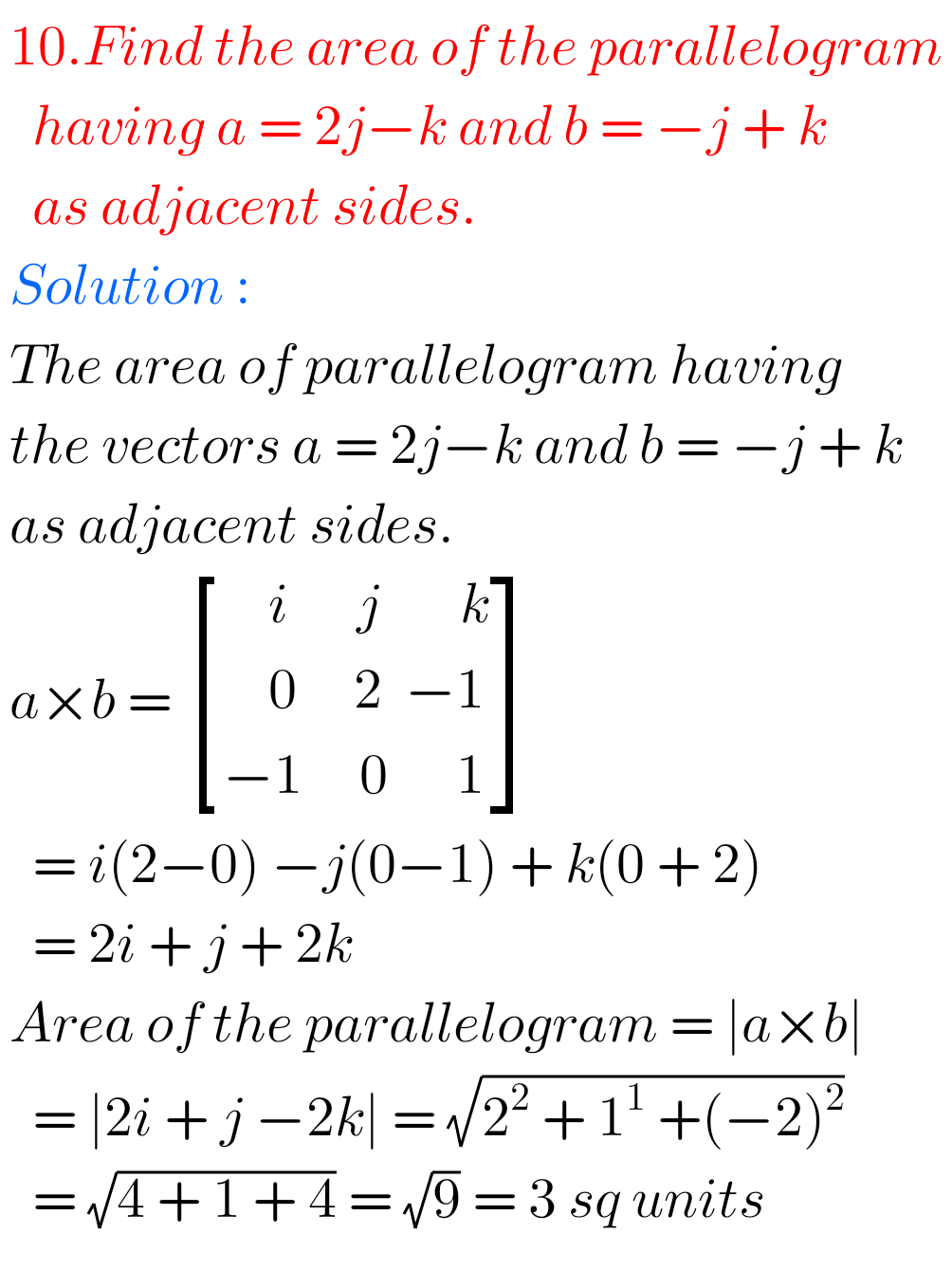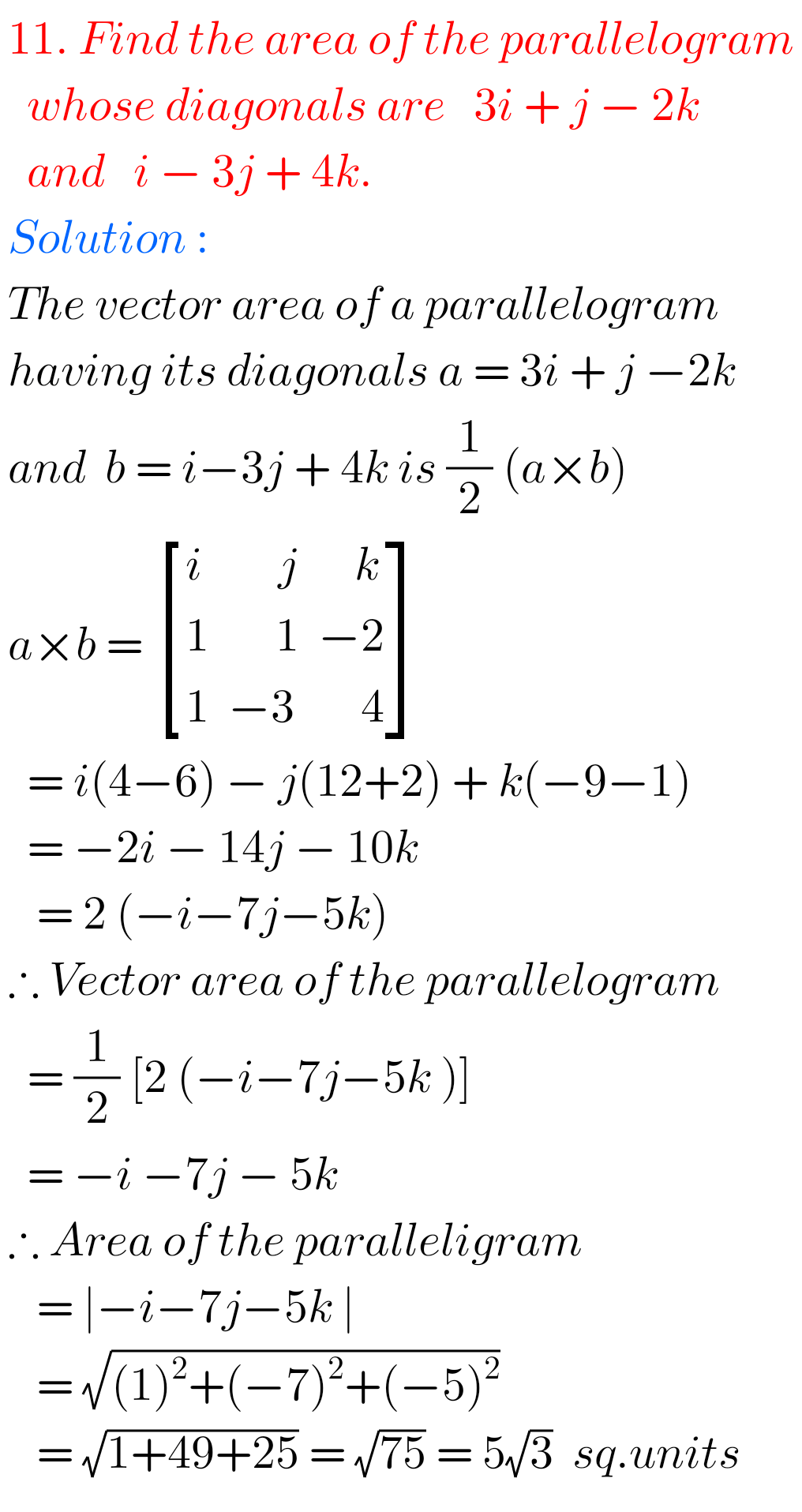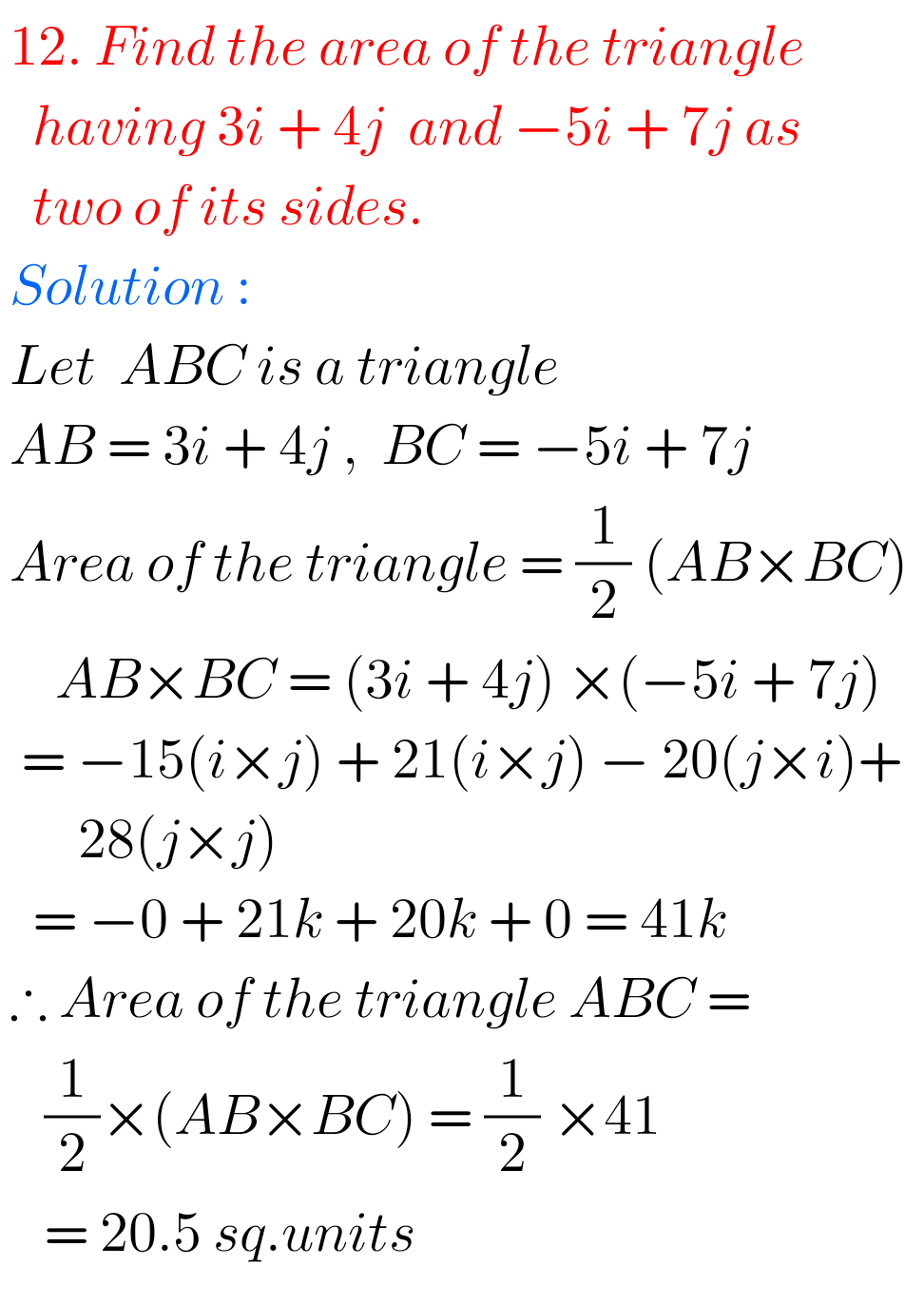## Maths Solutions for Product of Vectors Exercise 5(b)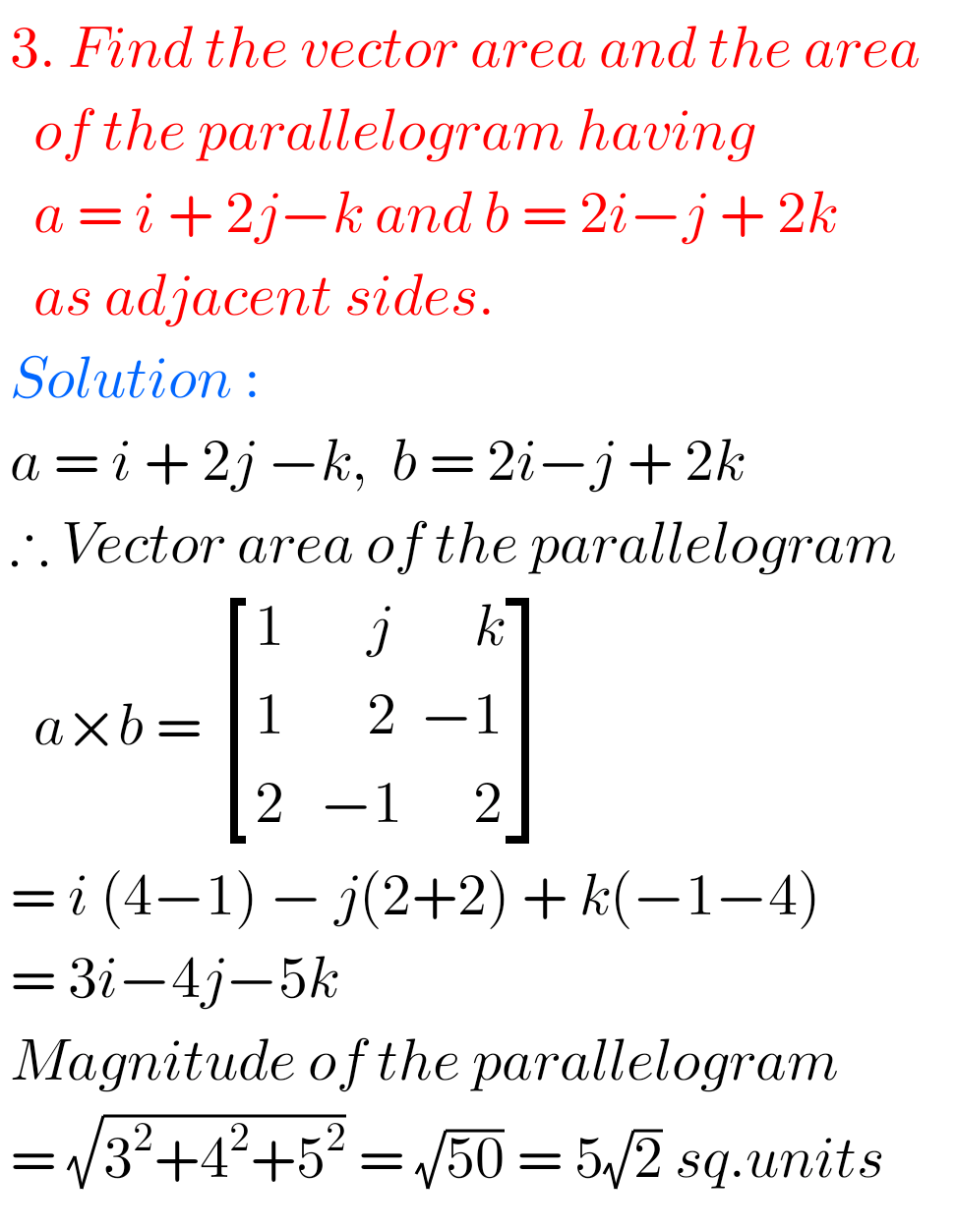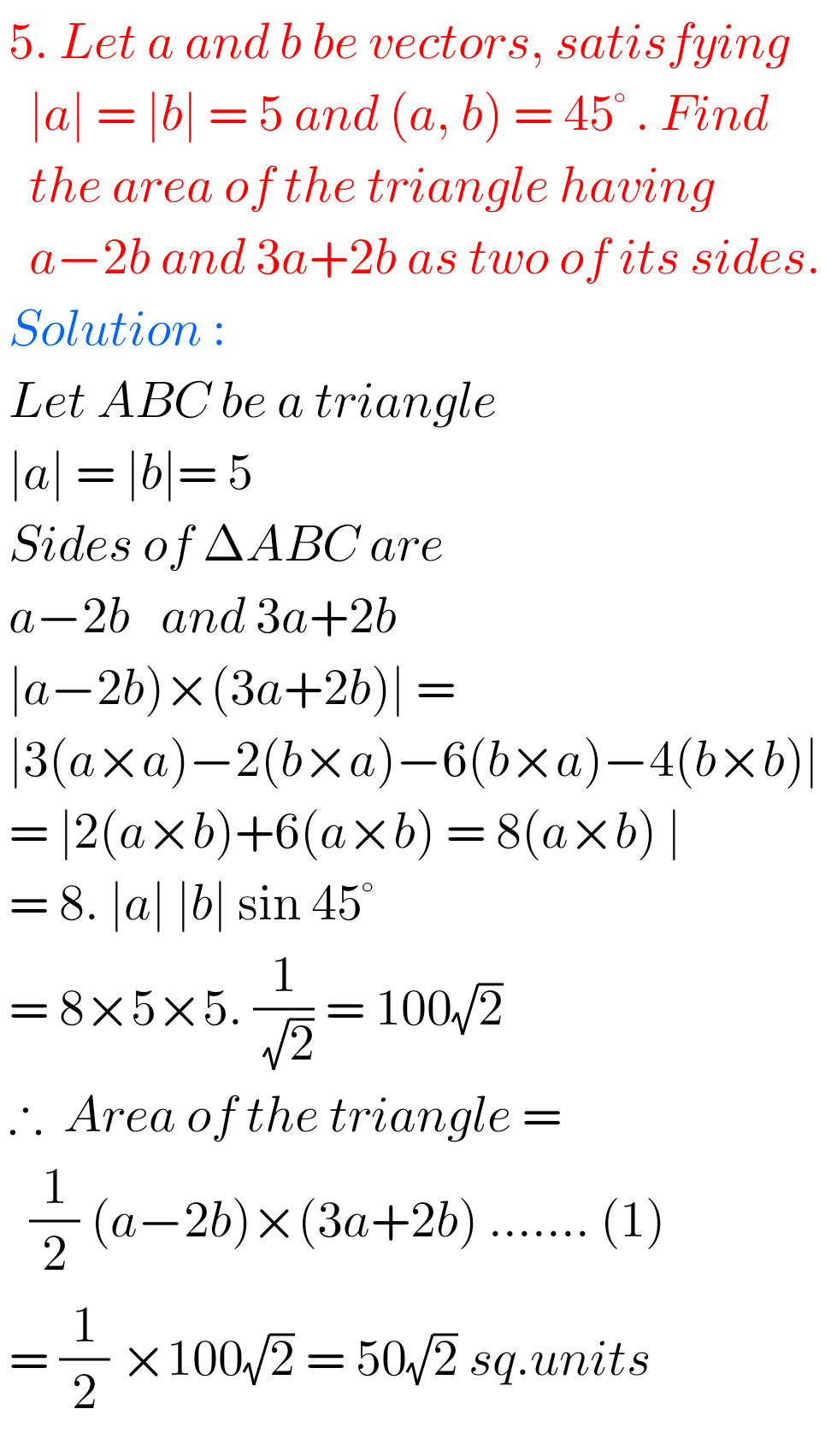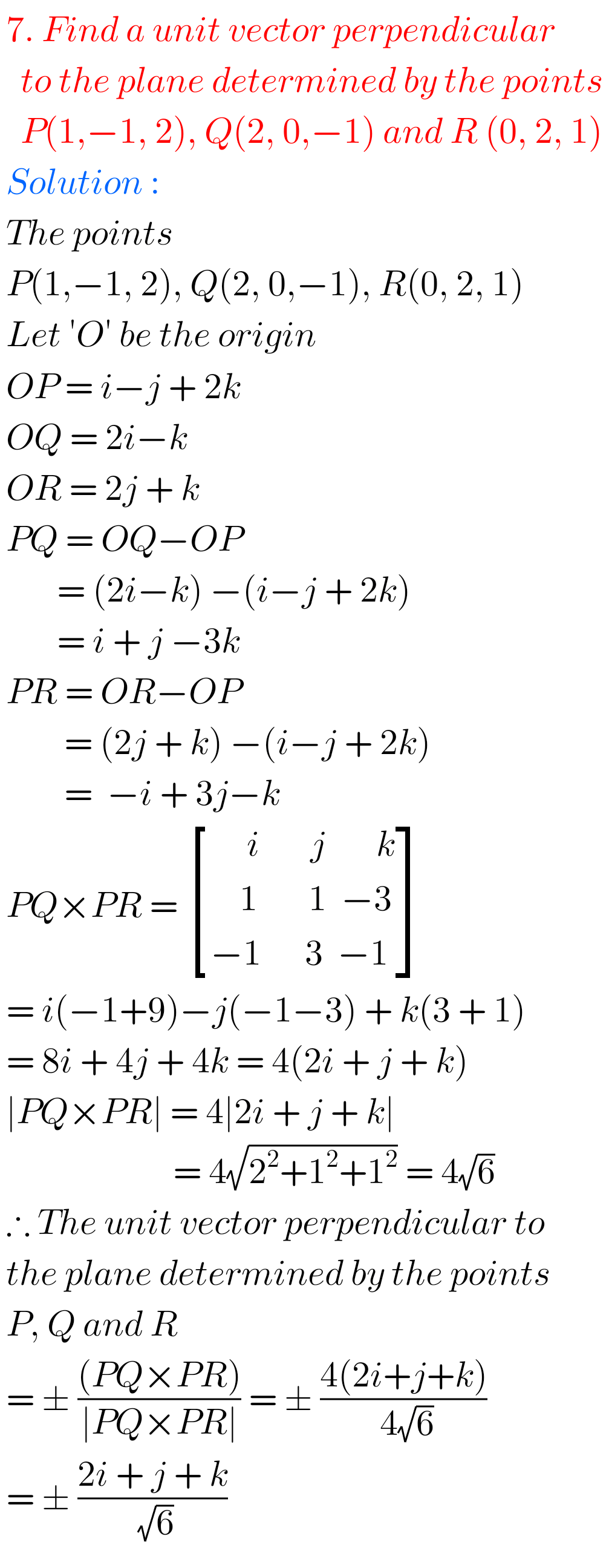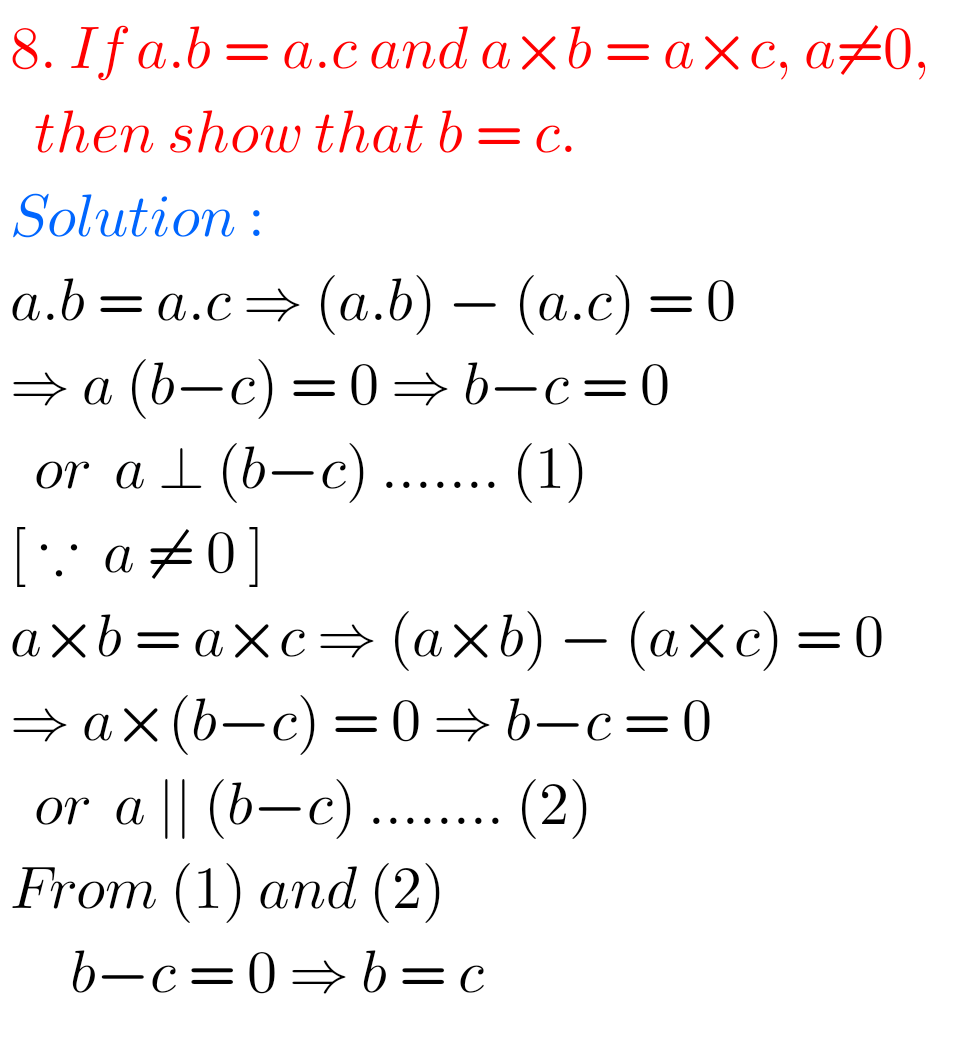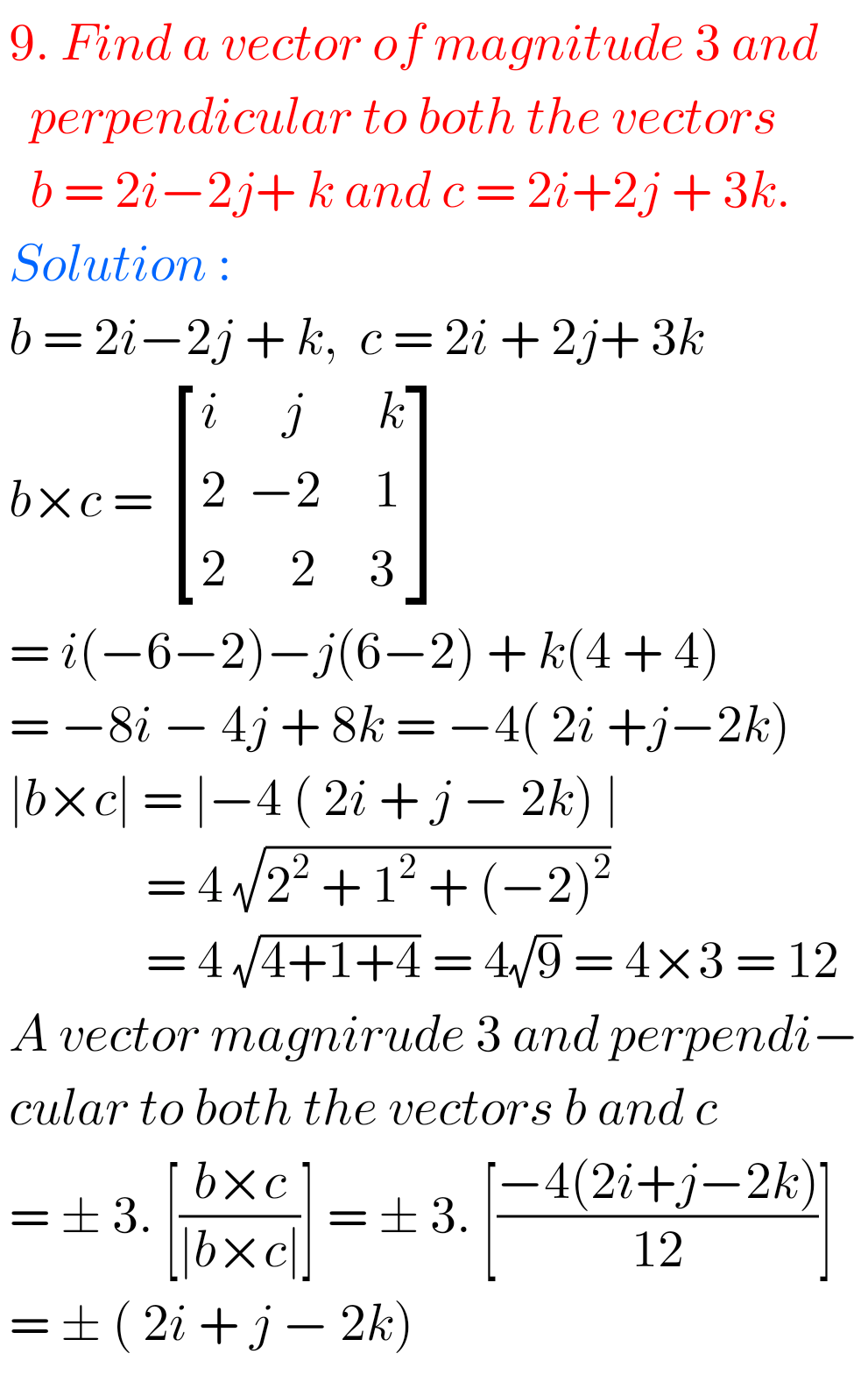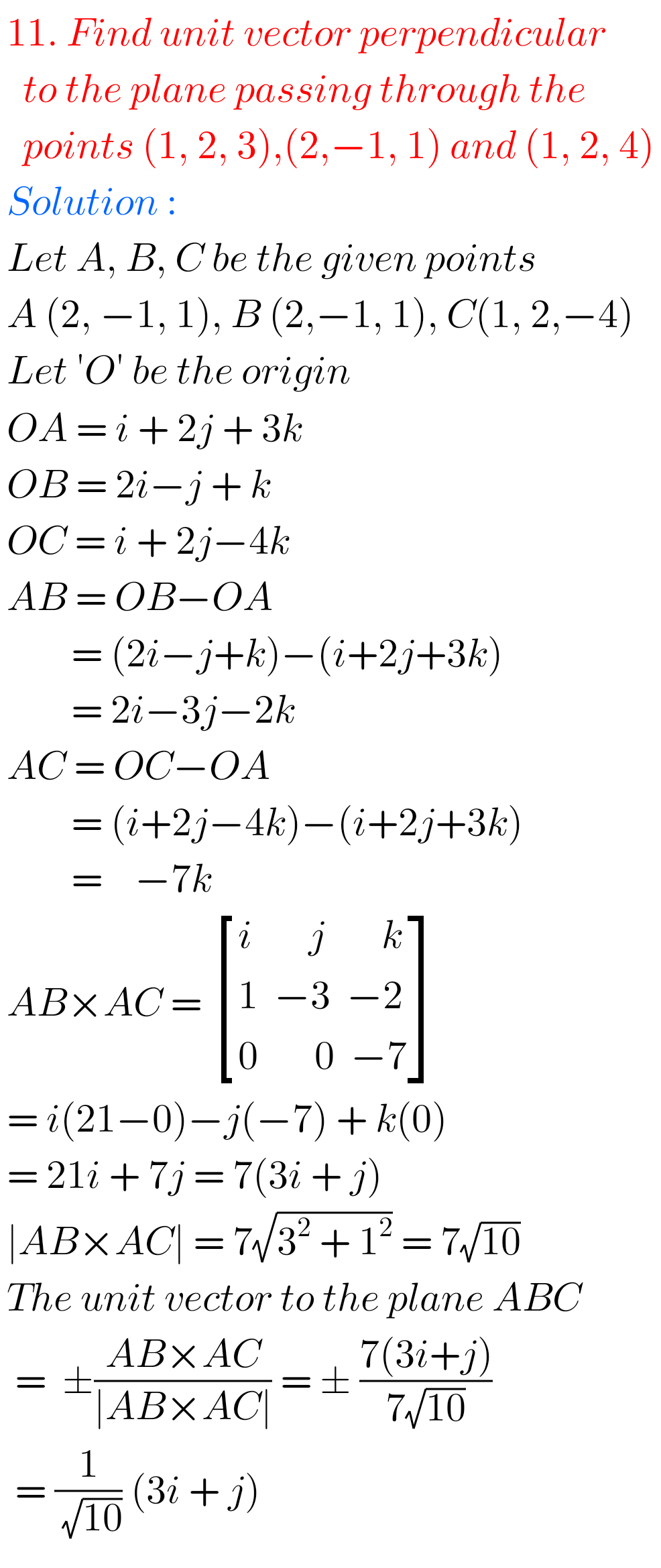## Product of Vectors Exercise 5(b) Solutions Inter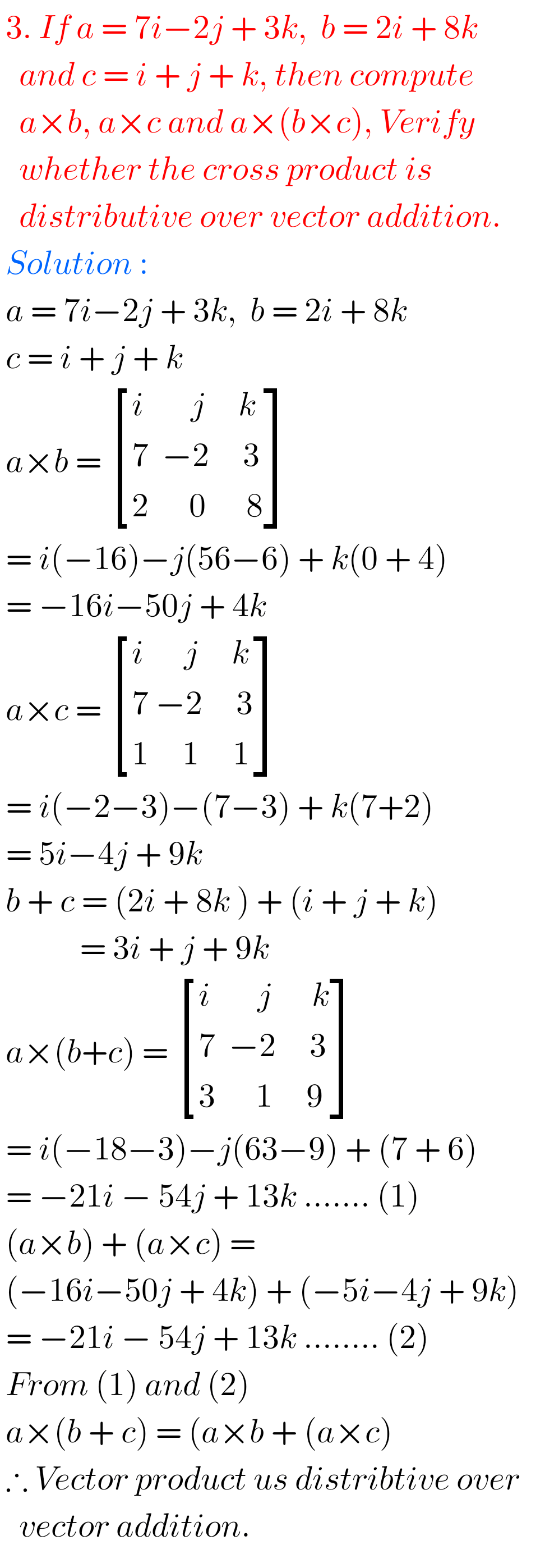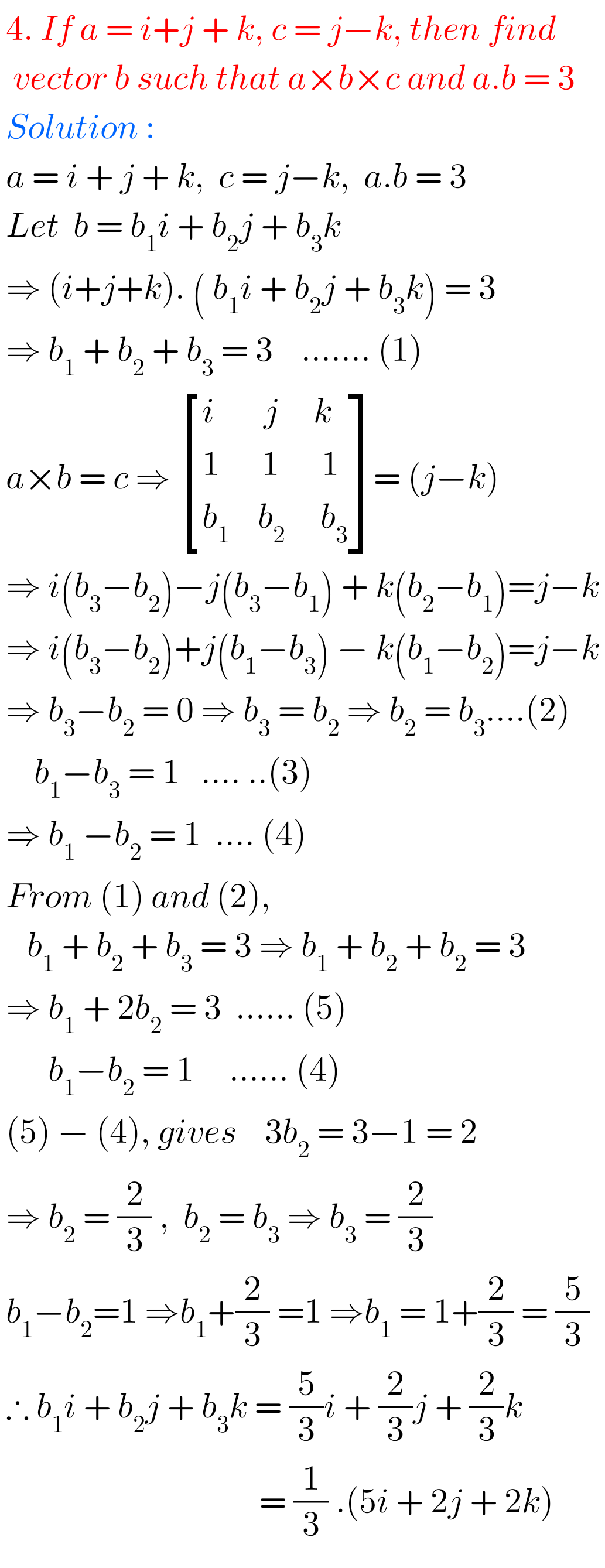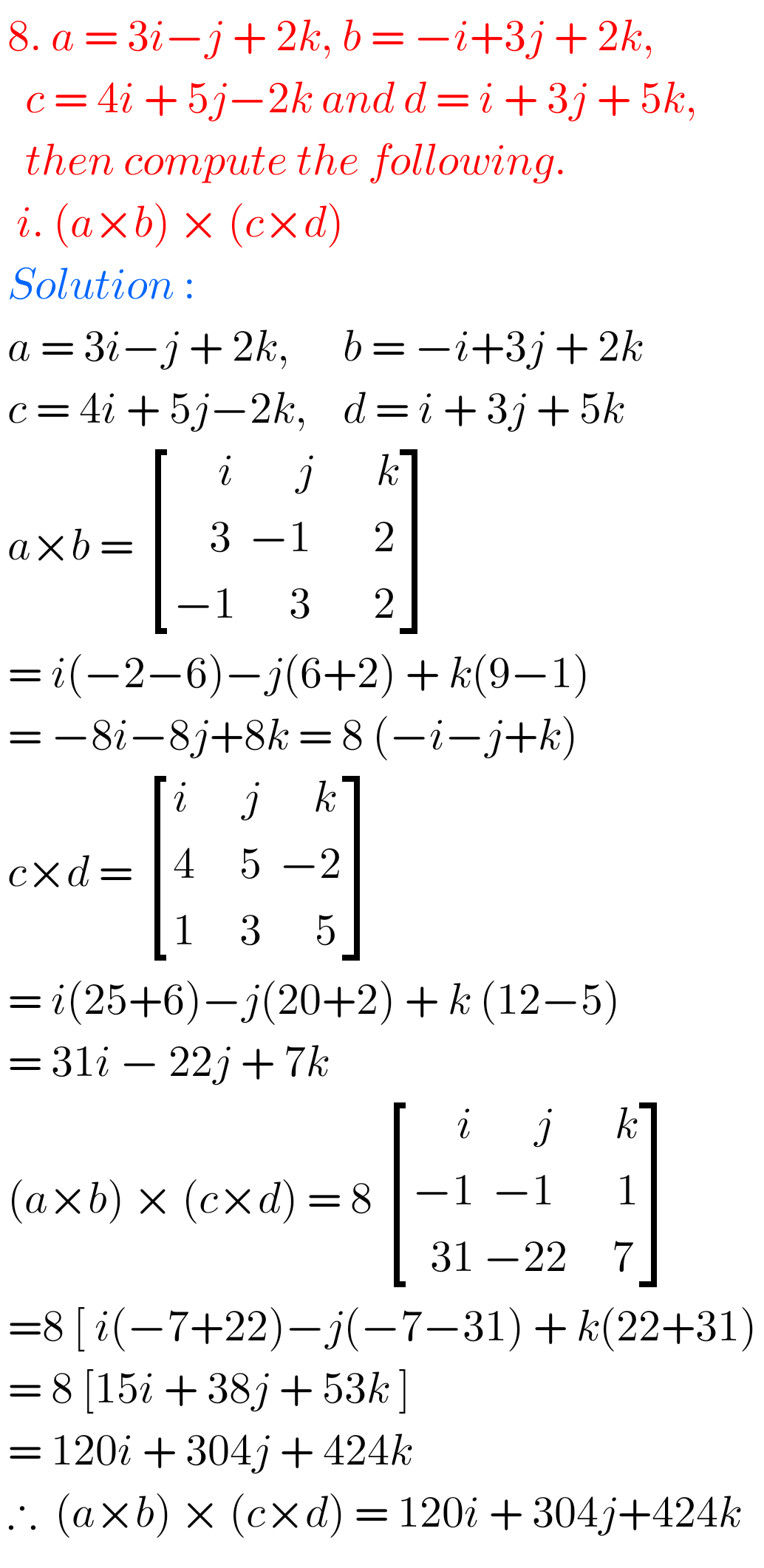Note : Observe the solutions and try them in your own method.

SSC Maths text book Solutions class 10

Inter Maths 1A solutions

Inter Maths 1B solutions

Inter Maths 1IA text book solutions

Inter Maths IIB text book solutions

You can see the solutions for junior inter maths 1A for examination purpose

2. Matrices

Junior inter 1B solutions of Examination purpose

1Locus

Straight lines sa

Straight lines la

12. Rate measure